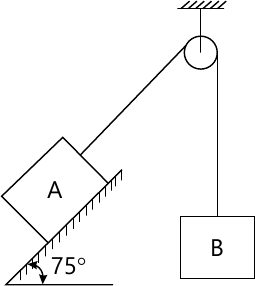# Engineering Mechanics Question paper December 2015

Engineering Mechanics Question paper December 2015 – The First year question paper for engineering mechanics for Mumbai university students of F.E. is given below:

## Engineering Mechanics Question paper December 2015

1 (a) Three concurrent forces P=150N, Q=250N and S=300N are acting at 120° with each other. Determine their resultant force magnitude and direction with respect to P. What is their equilibrant?
4 M
1 (b) A prismatic bar AB of length 6m and weight 3KN is hinged to a wall and supported by cable BC. Find hinge reaction and tension in cable BC.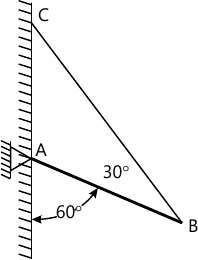4 M
1 (c) A block of weight 800N is acted upon by a horizontal force P as shown in figure. If the coefficient of friction between the block and incline are ?s=0.35 and ?k=0.25, detemine the value of P for impending motion up the plane.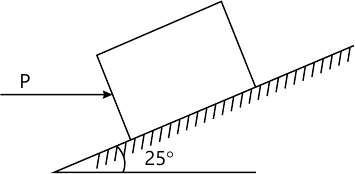4 M
1 (d) A bolt air balloon starts rising vertically up from the ground with an acceleration of 0.2 m/s2. 12 seconds later the man sitting inside the balloon releases a stone. Find the time taken by the stone to hit the ground.
4 M
1 (e) A small block rests on a turn table, 0.5m away from its centre. The turn table, starting from rest, is rotated in such a way that the block undergoes a constant tangential acceleration. Determine the angular velocity of the turn table at the instant when the block start slipping. ?=0.4.
4 M
2 (a) Three right circular cylinder A, B, C are piled up in a rectangular channel as shown in figure. Determine the reactions at point 6 between the cylinder A and vertical wall of the channel.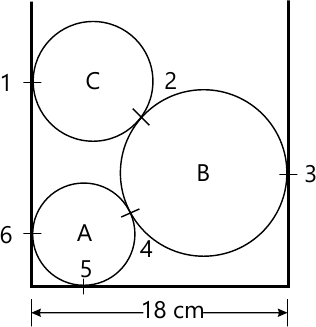8 M
2 (b) Four forces and a couple are acting on a plate as shown in figure. Determine the resultant force and locate it with respect to point A.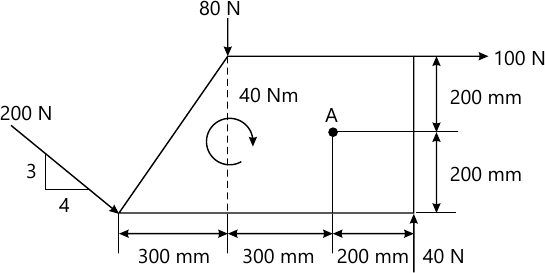6 M
2 (c) Two balls with masses 20kg and 30 kg are moving towards each other with velocities 10 m/s and 5m/s respectively. If after impact the ball having mass 30kg reverses its direction of motion and moves with velocity 6m/s, then determine the coefficient of restitution between the two balls.
6 M
3 (a) Determine the Centroid of the shaded area. All dimensions are in mm.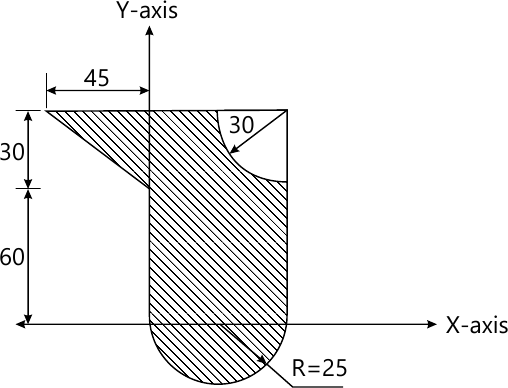8 M
3 (b) Force 5KN is acting along AB where A(0, 0, -1)m and B(5, -2, -4)m. Another force 8KN is acting along BC where C (3, 3, 4)m. FInd resultant of two forces and find moment of resultant force about a point D(0, 3, -2)m.
6 M
3 (c) A 2kg collar M is attached to a spring and slides without friction in a vertical plane along the curved rod ABC as shown in figure. The spring has an un-deformed length of 100mm and its stiffness k=800N/m. If the collar is released from rest at A, determine its velocity
i) as it passes through B
ii) as it reaches C.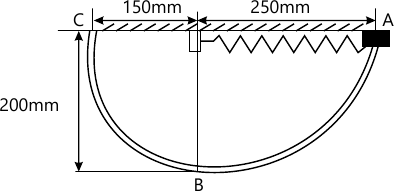6 M
4 (a) Find support reactions at A and B for the beam loaded as shown m figure. A is hinged and B is roller.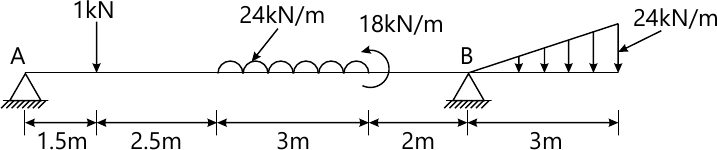8 M
4 (b) An object is projected so that it just clears two obstacles each of 7.5m height, which are situated 50 m from each other. If the time of passing between the obstacles is 2.5 s, calculate the complete range of projection and the initial velocity of the proctile.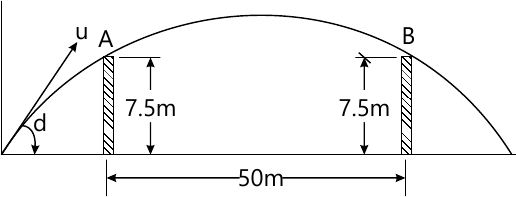6 M
4 (c) The crank BC of a slider crank mechanism is rotating at constant speed of 30 rpm clockwise. Determine the velocity of the piston A at the given instant.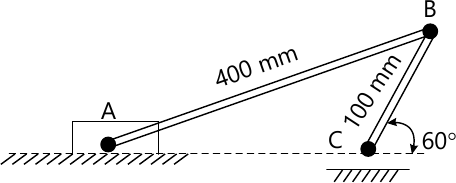6 M
5 (a) For the truss shown in figure, determine,
i) Support Reactions
ii) Forces in members CE and CF by method of sections only.
iii) Forces in any other members by method of joints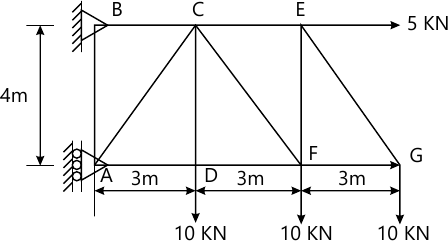8 M
5 (b) A particle moves in straight line with acceleration time diagram shown in figure. Construct velocity-time diagram for the motion assuming that the motion starts with initial velocity of 5m/s from the starting point. Also determine its displacement at t=12 seconds.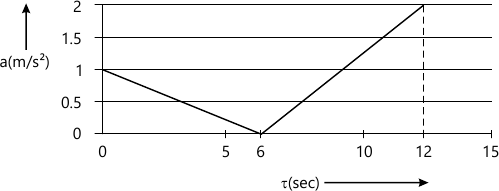6 M
5 (c) Due to slipping, points A and B on the rim of the disk have the velocity Va=1.5 m/s to the right and Vb=3m/s to the left as shown in figure. Determine the velocities of the centre point C and point D on the rim at this instant. Take radius of disk 0.24 m.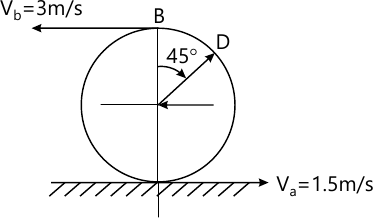6 M
6 (a) Find force requires to pull block B as shown. Coefficient of friction between A and B is 0.3 and between B and floor is 0.25. Mass of A=40kg and B=60kg.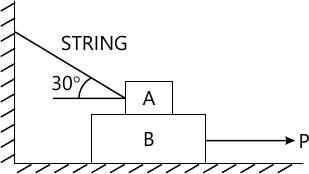8 M
6 (b) A force acts at the origin in a direction defined by the angles ?y=65° and ?z=407deg; Knowing that that the x-component of the force is -750N, determine
i) the other components
ii) magnitude of the force
iii) the value of ?x.
4 M
6 (c) A particle travels on a circular path, whose distance travelled is defined by S=(0.5t3+3t)m. If the total acceleration is 10 m/s2, at t=2 sec, find its radius of curvature.
4 M
6 (d) Block A and B of mass 6kg and 12kg respectively are connected by a string passing over a smooth pulley. Neglect mass of pulley. If coefficient of kinetic friction between the block A and the inclined surface is 0.2, determine the acceleration of block A and block B.